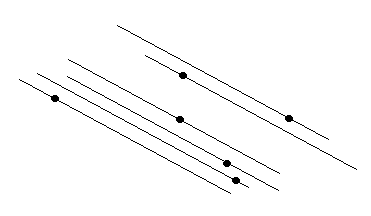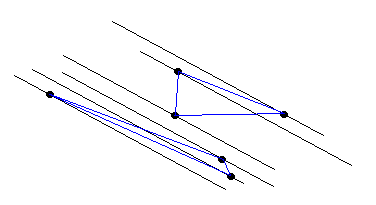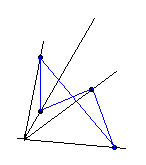## Partition of Point Sets in the Plane

### Problem

There are 3n points in the plane no three of which lie on the same straight line. Is it possible to form n triangles with vertices at these points so that the triangles have no points in common?

### Solution

Assuming the problem solved, we would have n triangles with no common points. The triangles are separated from each other so that between any two it would be possible to draw a line such that the triangles would lie on opposite sides of the line. Assuming also we know to draw such lines for any pair of the triangles so that no point from the given set lies on any of the lines, then those lines would partition the plane into regions with the property that any such region either contains exactly three points from the given set or contains no points at all.

If we could draw lines like these that separate the set into subsets of 3 points we would be able to solve the problem. So let us think of how can we draw these lines.

First of all, let us draw lines that we could not use for the stated purpose. Let us draw a line through every pair of points out of the given set. Depending on the magnitude of n, the plane will be more or less colored. Regardless, there will be plenty of room left. Also, we can easily pick up a direction different from (not parallel to) any of our lines. Let us draw a line in this direction away from the given set so that all 3n points lie on one side from that line.Let start moving the line towards the set keeping it parallel to the selected direction. After a while it will cross one of the given points. Note that lines in the selected direction may at most contain 1 point out of the given set. Continue moving the line until it intersects another point and so on. It appears that we can select a direction and draw lines in this direction such that every line contains one and only one point from the set.Now the solution becomes obvious. Admittedly, the lines are not what we've been looking for. However, they do the separation job quite nicely for the set of the lines is discrete. Select the first three and draw a triangle with vertices at points that lie on the selected lines. Pick up the next three lines, etc. Obviously, the triangles will not intersect.

What questions may we ask concerning the problem and, perhaps, the solution? What are parameters of the problem? 3n points. n being an arbitrary number anyway, let try changing the number 3:

There are 4n points in the plane no three of which lie on the same straight line. Is it possible to form n quadrilaterals with vertices at these points so that the quadrilaterals have no points in common?

Perhaps we can go a little further and pose the following problem:

There are N*n points in the plane no three of which lie on the same straight line. Is it possible to form n N-gons with vertices at these points so that the N-gons have no points in common?Obviously the solution extends to any number N of vertices. Just split the set of N*n parallel lines into subsets of N consecutive lines. Then draw the sides of the N-gons. Now we did it - solved a given problem and have also found that our solution works in a more general case. Good.

But does not something look wrong on the diagram? Yes, indeed. We were so anxious to find not intersecting polygons that somehow it seems weird to allow self-intersecting polygons, right? Let then reformulate our generalization:

There are N*n points in the plane no three of which lie on the same straight line. Is it possible to form n (non self-intersecting) N-gons with vertices at these points so that the N-gons have no points in common?

Let try to preserve a part of our solution. After all, it served us once and was helpful later on. We already know how to separate N*n points into subsets of N points that look very much as good candidates to be vertices of the sought polygon. If only we could solve the following problem:

There are N points in the plane no three of which lie on the same straight line. Is it possible to form an N-gon with vertices at these points so that no two of its sides intersect?

Looking at the last diagram it appears that one way or another we can handle the case of N=4. But, as N grows, we are bound to get into a mess unless, of course, we find a solution to our general problem.Again let us apply this approach that helped us previously. Let us again draw lines through every pair of the points out of the set of N points. As we noticed before, there is plenty of room left in the plane. We can definitely find a point that does not belong to any of the lines we drew. Let us draw rays (half-lines) through each of the given points and the one just selected. It appears that now we can connect consecutive points so that no two segments may possibly intersect. In fact, if every segment connecting two consecutive points remains inside a single sector formed by two consecutive rays, it can't possibly intersect any other segment with the same property.However, care must be exercised to insure that every such segment lies in a single sector. Can we achieve this? Yes, we can. What is necessary is to select our ray center in such a way that going from point to point we eventually would circle around the point selected. It somehow must be selected inside the given point set.

Return to our parallel lines and start, as before, with the one away from the set. Keeping it parallel to itself move it again until it meets the first point from the set. Now rotate it around this point until it meets another point from the set. Continue rotating it around the sequence of points obtained this way. Eventually we'll find what's called the convex hull of the given set of points - a convex polygon that has all the points of the given set as its vertices with some of them strictly in its interior. Selecting our ray center anywhere inside the convex hull of the given set of N points will solve the problem.